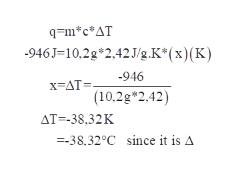# A 10.2−g sample of ethylene glycol, a car radiator coolant, loses 946 J of heat. What was the initial temperature of the ethylene glycol if the final temperature is 32.5°C? (c of ethylene glycol = 2.42 J/g·K)what is the answer in Chelp

Question
2 views

A 10.2−g sample of ethylene glycol, a car radiator coolant, loses 946 J of heat. What was the initial temperature of the ethylene glycol if the final temperature is 32.5°C? (c of ethylene glycol = 2.42 J/g·K)

what is the answer in C

help

check_circle

Step 1

Given:

Mass of ethylene glycol=10.2 g

Heat loss (q)=946 J

Final temperature=32.5oC

Specific heat capacity (c) of ethylene glycol=2.42 J/g.K

Step 2

To calculate initial temperature of ethylene glycol:

Consider an equation, where heat flow (q) of process is equal to change in enthalpy

Step 3

Calculating the initial temperature,

By substituting all t...help_outlineImage Transcriptioncloseq=m*c*AT -946J-10,2g*2,42J/g.K* ( x) (K) 946 x AT (10,2g 2.42) AT--38.32K 38.32°C since it is A fullscreen

### Want to see the full answer?

See Solution

#### Want to see this answer and more?

Solutions are written by subject experts who are available 24/7. Questions are typically answered within 1 hour.*

See Solution
*Response times may vary by subject and question.
Tagged in

### Physical Chemistry